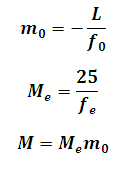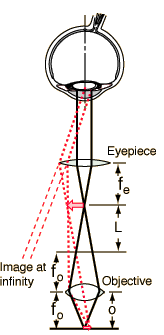# Microscope Magnification Calculator

This Calctown Calculator calculates the magnification of compound microscope.

cm
mm
mm

#### Resultwhere

L = tube length

f0 = objective focal length

fe = eyepeice focal length

m0 = linear magnification of objective

Me = angular magnification of eyepeice

M = total magnification of microscope